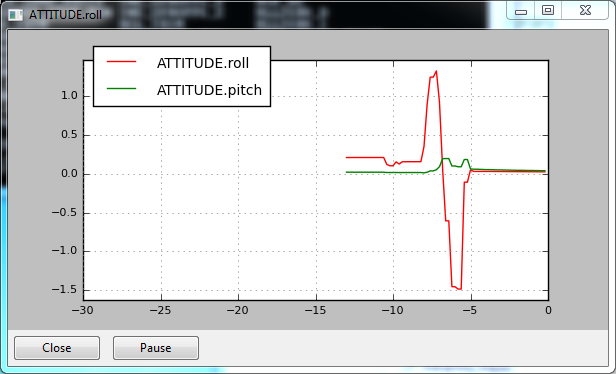# Graphing Live Data¶

```module load graph
```

A module to plot (graph) data in realtime from the UAV. It is useful for looking for time-varying patterns in the data

```graph dataname
```

Multiple items can be added at once. Use the `:2` to specify using the right vertical axis.

```graph VFR_HUD.alt VFR_HUD.airspeed:2
```

Arbitrary mathematical functions can also be used.

```graph "(VFR_HUD.alt/1000.0)+5"
```

Additionally, the timespan and tick resolution of the graph window can be changed. Use `graph timespan X` to change the timespace to X seconds and `graph tickresolution Y` to change the tick resolution to Y seconds.There are two global settings for graphs: timespan and tickresolution.

To set the timespan along the horizontal axis (in seconds):

```   graph timespan 20

To set the tickresolution along the horizontal axis (1/<number of ticks>):
```
```graph timespan 0.2
```